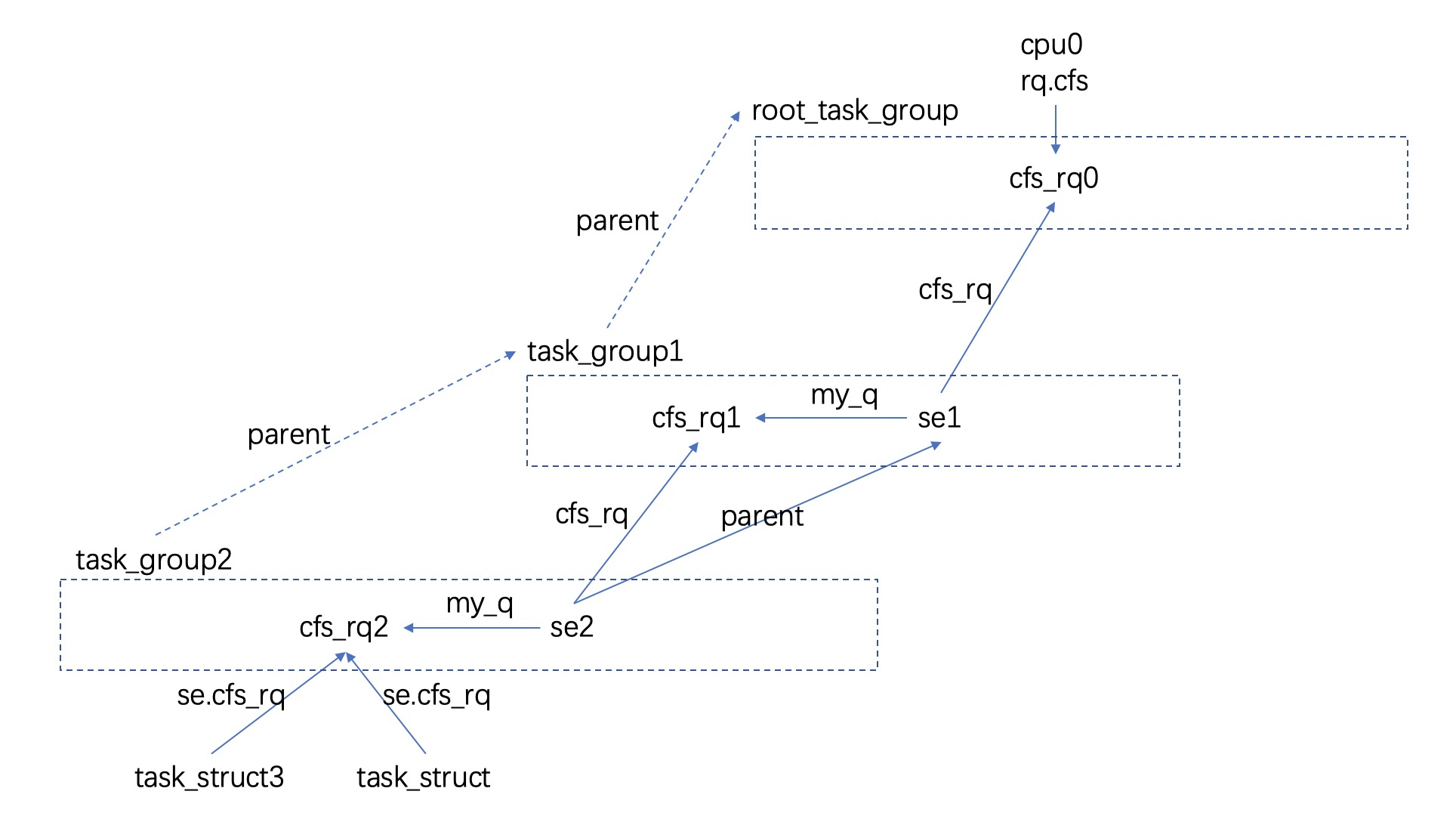# linux 中的 pelt

Posted by w@hidva.com on January 13, 2022${w_n * t_n * y ^ n + w_{n-1} * t_{n-1} * y ^ {n-1} + ... + w_1 * t_1 * y ^ 1 + w_0 * t_0 * y ^ 0 } \over { W * y ^ n + W * y ^ {n-1} + ... + W * y ^ 1 + W * y ^ 0 }$

$w_n * {t_n \over W} * y ^ n + w_{n-1} * {t_{n-1} \over W} * y ^ {n-1} + ... + w_1 * {t_1 \over W} * y ^ 1 + w_0 * {t_0 \over W} * y ^ 0$

• runnable_avg, 此时若 task_struct 处于可运行/正运行的状态时, w 的值是 1; 若 task_struct 处于不可运行时, w = 0.
• util_avg, 此时若 task_struct 处于正运行的状态时, w 的值是 1; 其他情况下时, w = 0.

### util_avg V.S. cpu capacity

The load/runnable/util_avg doesn’t directly factor frequency scaling and CPU capacity scaling. The scaling is done through the rq_clock_pelt that is used for computing those signals (see update_rq_clock_pelt())

### 何时更新

1. se1 在时刻 t1 被从正运行状态切走, se1 pelt 信息刚在上一次 tick 时 t0 更新, 此时调用链 __schedule() –> pick_next_task()/pick_next_task_fair() –> put_prev_task()/put_prev_task_fair() –> put_prev_entity() –> update_load_avg(cfs_rq, prev, 0); 此时在 update_load_avg() 调用时, se1.cfs_rq.curr 仍然是 se1, 即在 update_load_avg 视角 se1 仍然是 running 状态. 此时时间窗口为 [se.avg.last_update_time=t0, now=t1], 这里 update_load_avg 调用会补齐 [t0, t1] 这段窗口内 se pelt 信息.
2. 这之后 se1 不再是 running 状态, 其 pelt 一直不被更新, se.avg.last_update_time 永远停留在 t1.
3. 在 t2 时刻重新调度 se1 到正运行状态, 此时调用链 __schedule() –> pick_next_task()/pick_next_task_fair() –> set_next_entity() –> update_load_avg(), 这里 update_load_avg 调用时, cfs_rq.curr 尚不是 se1, 在 update_load_avg 看来 se1 仍不是 running 状态; update_load_avg 补齐了 [t1, t2] 这段窗口内 se pelt 信息.

## cfs_rq pelt

cfs_rq.avg 存放着 cfs_rq 粒度的 pelt 信息, cfs_rq pelt 信息等于其内所有 se, 包含正运行的, 可运行的, 不可运行的, pelt 加在一起的结果. 如文章最开始摆放的 cfs_rq 层次图: cfs_rq2.pelt = task_struct3.pelt + task_struct.pelt. 关于 cfs_rq pelt 的更新, 一种最糙的法子是每 1024us 针对每一个 cfs_rq, 计算其内所有 se pelt, 之后累加起来作为 cfs_rq pelt, 很明显 linux 不会采用这种方法, 但最终实现了一样的效果. 在每次 tick 更新 running se pelt 时, 会连带着更新 cfs_rq pelt, 此时 cfs_rq pelt 计算与 se pelt 计算公式一样, 主要在与 w 值不同:

• runnable_avg, w = cfs_rq.h_nr_running, h_nr_running 为所有可运行, 正运行 se 的数目.
• util_avg, w = cfs_rq.curr != NULL, cfs_rq.curr != 0 意味着当前 cpu 正运行的 se 是 cfs_rq.curr.

1. 在时刻 t0, cfsrq1 中有三个 se se1, se2, se3, se1 正运行, se2, se3 可运行. 此时在 tick 中计算 cfsrq1 pelt, 这里 contrib 见 accumulate_sum(), divider 见 ___update_load_avg():

$cfsrq1.pelt.t0 = cfsrq1.weight * {contrib \over divider } \\= (se1.w + se2.w + se3.w) * {contrib \over divider } \\= (se1.w * {contrib \over divider }) + (se2.w * {contrib \over divider }) + (se3.w * {contrib \over divider }) \\=se1.pelt.t0 + se2.pelt.t0 + se.pelt.t0$
2. 在时刻 t1, se1 sleep, 切换到 se2 运行, 此时在 tick 中计算 cfsrq1 pelt:

$cfsrq1.pelt.t1 = cfsrq1.pelt.t0 * y ^ 1 + cfsrq1.weight * {contrib \over divider } \\=cfsrq1.pelt.t0 * y ^ 1 + (se2.w + se3.w) * {contrib \over divider } \\=se1.pelt.t0 * y ^ 1 \\+ se2.pelt.t0 * y ^ 1 + se2.pelt.t1 \\+ se3.pelt.t0 * y ^ 1 + se3.pelt.t1$

由于 se1 blocked, 即 se1.pelt.t1 被认为是 0, 但 se1.pelt.t0 仍然在 cfsrq1.pelt 中, 一起被衰减了. 这也是 pelt 主张的: blocked se 仍然是有负载贡献的.

## group se pelt

group se pelt 等于其 my_q 的 pelt 信息, 即: se2.pelt = se2.my_q.pelt. task_group.pelt = 其内所有 cfs_rq pelt 之和. 另外 group se weight = task_group.shares * (gse.load_avg / tg.load_avg). 这些设定看起来还是很合理的. group se, task group pelt, group se weight 都是在 tick 更新 running se pelt 时自底向上完成更新. 如下以 task_struct3 迁入当前 cpu 执行场景为例, 此时与 pelt 相关链路:

1. enqueue_entity(cfs_rq2, se3);
1. 此时 se3 是迁入, 其 last_update_time 为 0, attach_entity_load_avg(cfs_rq2, se3)
1. cfs_rq2.propagate = 1;
2. enqueue_entity(cfs_rq1, se2);
1. cfs_rq1.propagate = 1; cfs_rq1.prop_runnable_sum = cfs_rq2.prop_runnable_sum.
2. update_tg_cfs_util(cfs_rq1, se2, cfs_rq2);
3. update_tg_cfs_runnable(cfs_rq1, se2, cfs_rq2)
3. enqueue_entity(cfs_rq0, se1)
1. add_tg_cfs_propagate(cfs_rq0, cfs_rq1.prop_runnable_sum); cfs_rq0.propagate = 1, cfs_rq0.prop_runnable_sum = cfs_rq1.prop_runnable_sum
2. update_tg_cfs_util(cfs_rq0, se1, cfs_rq1);
3. update_tg_cfs_runnable(cfs_rq0, se1, cfs_rq1)